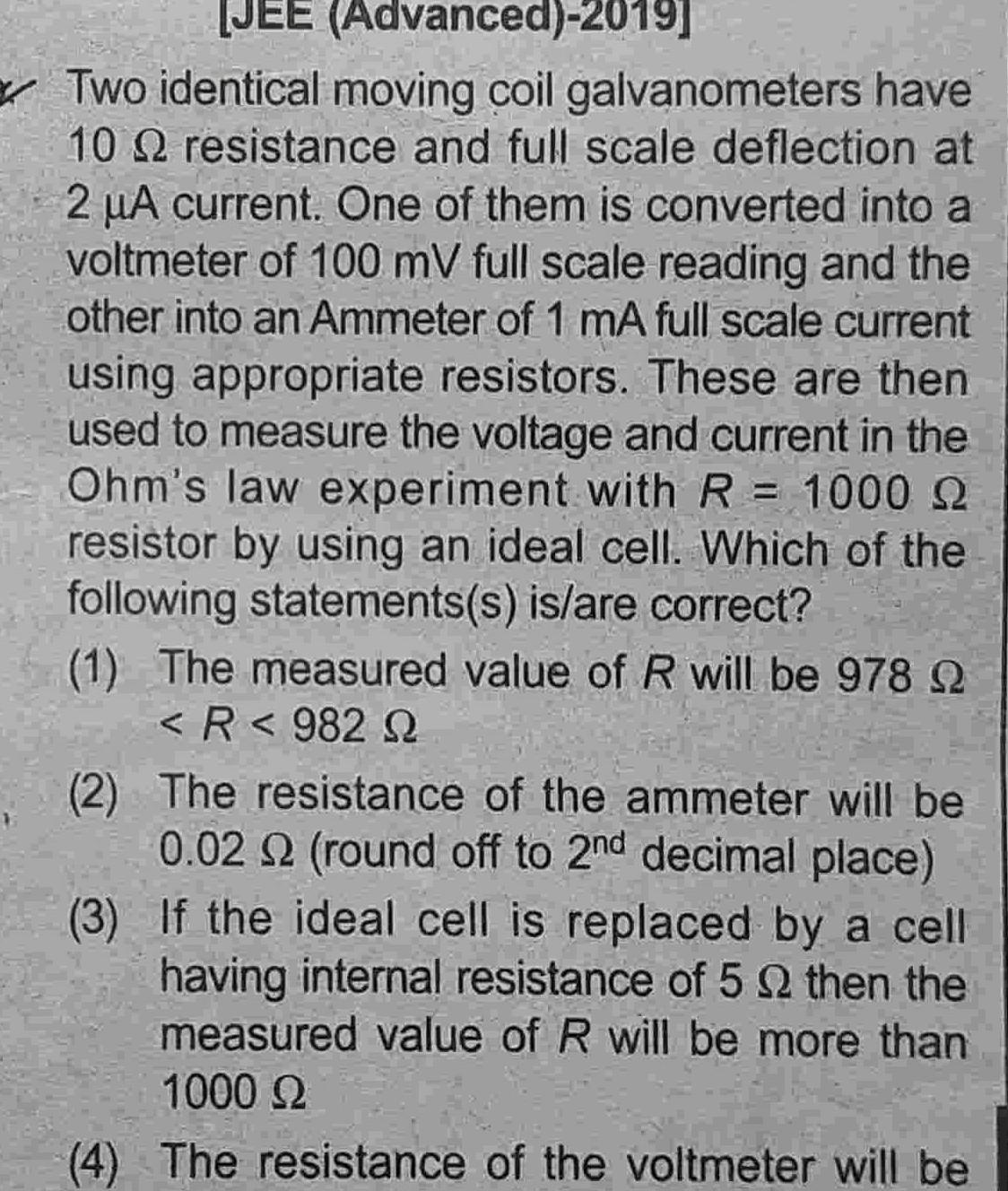Question:

# JEE Advanced 2019 Two identical moving coil galvanometers

Last updated: 6/26/2023JEE Advanced 2019 Two identical moving coil galvanometers have 10 resistance and full scale deflection at 2 A current One of them is converted into a voltmeter of 100 mV full scale reading and the other into an Ammeter of 1 mA full scale current using appropriate resistors These are then used to measure the voltage and current in the Ohm s law experiment with R 1000 22 resistor by using an ideal cell Which of the following statements s is are correct 1 The measured value of R will be 978 9 R982 Q 2 The resistance of the ammeter will be 0 02 2 round off to 2nd decimal place If the ideal cell is replaced by a cell having internal resistance of 5 2 then the measured value of R will be more than 1000 S 3 4 The resistance of the voltmeter will be